###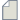Author Topic: Plotting the Eigen state of a given structure  (Read 419 times)

0 Members and 1 Guest are viewing this topic.

####Shivam Das

• New QuantumATK user
•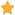• Posts: 4
• Country: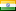• Reputation: 0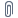##### Plotting the Eigen state of a given structure
« on: April 4, 2023, 17:38 »
I am using ATK 2019.03, for computing the eigenstate of a given structure, these are some questions I have regarding computation.
1) What should I put in the "Quantum number" attribute?
2) Do I have to check every option in the "Projection Selector"?
3) What should I include under the "Atom indices" attribute?
4) Do I have to check every orbital (s, p, d, f)?
Kindly help
check the attachment below

####filipr

• QuantumATK Staff
• Heavy QuantumATK user
•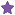• Posts: 60
• Country:• Reputation: 5
• QuantumATK developer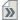##### Re: Plotting the Eigen state of a given structure
« Reply #1 on: April 13, 2023, 11:49 »
See https://docs.quantumatk.com/manual/Types/Eigenstate/Eigenstate.html for reference.

• "Quantum number" is in this context simply the index of the state you want to calculate and plot. The index 0 corresponds to the state with the lowest energy.
• You typically don't have to check any of the projection settings - only if you know you have to
• "Atom indices" is a subsetting under "Projection Selector", so unless you want to project the Hamiltonian on a subsystem, you also should leave this blank
• Same as above

So just put in a number in "Quantum number", leave rest blank and you should be fine. Note that "Eigenstate" is for molecules only - if you want to visualize the eigenstates of an extended, periodic system you should use "Bloch state" instead.

####dprai

• Regular QuantumATK user
•• Posts: 22
• Country:• Reputation: 0
• Learning Simulation of Nanoelectronics##### Re: Plotting the Eigen state of a given structure
« Reply #2 on: April 15, 2023, 08:42 »
Dear Filipr,
What to do when we have a gas adsorbed on a periodic system?
How shall we perform the HOMO-LUMO calculation in such case?

####karan1234

• Regular QuantumATK user
•• Posts: 5
• Country:• Reputation: 0##### Re: Plotting the Eigen state of a given structure
« Reply #3 on: April 17, 2023, 06:16 »
You can run the simulation for molecular energy spectrum to get this information.

####filipr

• QuantumATK Staff
• Heavy QuantumATK user
•• Posts: 60
• Country:• Reputation: 5
• QuantumATK developer##### Re: Plotting the Eigen state of a given structure
« Reply #4 on: April 17, 2023, 09:09 »
Dear Filipr,
What to do when we have a gas adsorbed on a periodic system?
How shall we perform the HOMO-LUMO calculation in such case?

• You would have to ensure that the size of the periodic system is repeated enough times so that the molecule does not artificially interact with its periodic images in the neighboring cells. You can either just guesstimate the size/number of repeats or do a convergence study if you want to be more thorough.
• You can use a k-point sampling for the ground state calculation, of if the system is big enough it should be sufficient with a Gamma only calculation
• Then you calculate the BandStructure of the system.
• You need to find the band indices (= quantum numbers) of the states corresponding to the HOMO and LUMO on the molecule. Localized states on the molecule will typically have zero dispersion (if you made the system big enough), so they appear as a flat horizontal line in the band structure spectrum. It can be a bit hard to identify these on a spectrum where all the bands have been folded in due to the repeats of the substrate unit cell.
• Another approach is to calculate the Eigenvalues (or just use BandStructure) at the Gamma point and select a few states around the Fermi level , then calculate the BlochState for those and visually inspect whether they are localized on the molecule or not.